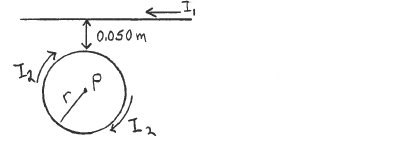Problem: A long straight wire carries current  I1 = 16.0 A and a circular loop of wire with radius  r = 0.200 m carries current I2 = 22.0 A. The two currents are in the directions shown in the sketch. The edge of the loop is 0.0500 m from the wire. What are the magnitude and direction of the resultant magnetic field at point P, which is at the center of the loop?

FREE Expert Solution
79% (449 ratings)
Problem Details

A long straight wire carries current  I1 = 16.0 A and a circular loop of wire with radius  r = 0.200 m carries current I2 = 22.0 A. The two currents are in the directions shown in the sketch. The edge of the loop is 0.0500 m from the wire. What are the magnitude and direction of the resultant magnetic field at point P, which is at the center of the loop?